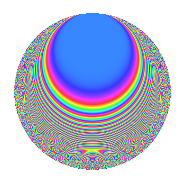# Properties

 Label 801.2.aLevel 801 Weight 2 Character orbit a Rep. character $$\chi_{801}(1,\cdot)$$ Character field $$\Q$$ Dimension 36 Newforms 11 Sturm bound 180 Trace bound 5

# Related objects

## Defining parameters

 Level: $$N$$ = $$801 = 3^{2} \cdot 89$$ Weight: $$k$$ = $$2$$ Character orbit: $$[\chi]$$ = 801.a (trivial) Character field: $$\Q$$ Newforms: $$11$$ Sturm bound: $$180$$ Trace bound: $$5$$ Distinguishing $$T_p$$: $$2$$, $$5$$

## Dimensions

The following table gives the dimensions of various subspaces of $$M_{2}(\Gamma_0(801))$$.

Total New Old
Modular forms 94 36 58
Cusp forms 87 36 51
Eisenstein series 7 0 7

The following table gives the dimensions of the cuspidal new subspaces with specified eigenvalues for the Atkin-Lehner operators and the Fricke involution.

$$3$$$$89$$FrickeDim.
$$+$$$$+$$$$+$$$$7$$
$$+$$$$-$$$$-$$$$7$$
$$-$$$$+$$$$-$$$$14$$
$$-$$$$-$$$$+$$$$8$$
Plus space$$+$$$$15$$
Minus space$$-$$$$21$$

## Trace form

 $$36q$$ $$\mathstrut +\mathstrut 2q^{2}$$ $$\mathstrut +\mathstrut 32q^{4}$$ $$\mathstrut +\mathstrut 2q^{5}$$ $$\mathstrut -\mathstrut 2q^{7}$$ $$\mathstrut +\mathstrut 6q^{8}$$ $$\mathstrut +\mathstrut O(q^{10})$$ $$36q$$ $$\mathstrut +\mathstrut 2q^{2}$$ $$\mathstrut +\mathstrut 32q^{4}$$ $$\mathstrut +\mathstrut 2q^{5}$$ $$\mathstrut -\mathstrut 2q^{7}$$ $$\mathstrut +\mathstrut 6q^{8}$$ $$\mathstrut -\mathstrut 2q^{10}$$ $$\mathstrut +\mathstrut 4q^{11}$$ $$\mathstrut -\mathstrut 6q^{13}$$ $$\mathstrut +\mathstrut 8q^{14}$$ $$\mathstrut +\mathstrut 24q^{16}$$ $$\mathstrut +\mathstrut 2q^{17}$$ $$\mathstrut -\mathstrut 14q^{19}$$ $$\mathstrut +\mathstrut 14q^{20}$$ $$\mathstrut +\mathstrut 4q^{22}$$ $$\mathstrut -\mathstrut 6q^{23}$$ $$\mathstrut +\mathstrut 18q^{25}$$ $$\mathstrut -\mathstrut 18q^{26}$$ $$\mathstrut +\mathstrut 14q^{28}$$ $$\mathstrut +\mathstrut 6q^{29}$$ $$\mathstrut -\mathstrut 8q^{31}$$ $$\mathstrut +\mathstrut 14q^{32}$$ $$\mathstrut -\mathstrut 2q^{34}$$ $$\mathstrut -\mathstrut 8q^{37}$$ $$\mathstrut +\mathstrut 10q^{38}$$ $$\mathstrut -\mathstrut 6q^{40}$$ $$\mathstrut -\mathstrut 10q^{41}$$ $$\mathstrut +\mathstrut 8q^{43}$$ $$\mathstrut +\mathstrut 32q^{44}$$ $$\mathstrut +\mathstrut 10q^{46}$$ $$\mathstrut +\mathstrut 20q^{47}$$ $$\mathstrut +\mathstrut 4q^{49}$$ $$\mathstrut -\mathstrut 28q^{50}$$ $$\mathstrut -\mathstrut 6q^{52}$$ $$\mathstrut +\mathstrut 34q^{53}$$ $$\mathstrut -\mathstrut 8q^{55}$$ $$\mathstrut -\mathstrut 8q^{56}$$ $$\mathstrut +\mathstrut 38q^{58}$$ $$\mathstrut -\mathstrut 10q^{59}$$ $$\mathstrut -\mathstrut 6q^{61}$$ $$\mathstrut -\mathstrut 14q^{62}$$ $$\mathstrut +\mathstrut 32q^{64}$$ $$\mathstrut -\mathstrut 30q^{65}$$ $$\mathstrut -\mathstrut 20q^{67}$$ $$\mathstrut +\mathstrut 2q^{68}$$ $$\mathstrut -\mathstrut 34q^{70}$$ $$\mathstrut -\mathstrut 12q^{71}$$ $$\mathstrut -\mathstrut 18q^{73}$$ $$\mathstrut -\mathstrut 12q^{74}$$ $$\mathstrut -\mathstrut 40q^{76}$$ $$\mathstrut +\mathstrut 28q^{77}$$ $$\mathstrut -\mathstrut 8q^{79}$$ $$\mathstrut +\mathstrut 42q^{80}$$ $$\mathstrut -\mathstrut 2q^{82}$$ $$\mathstrut -\mathstrut 10q^{83}$$ $$\mathstrut +\mathstrut 2q^{85}$$ $$\mathstrut +\mathstrut 52q^{86}$$ $$\mathstrut +\mathstrut 12q^{88}$$ $$\mathstrut -\mathstrut 6q^{89}$$ $$\mathstrut -\mathstrut 16q^{91}$$ $$\mathstrut -\mathstrut 44q^{92}$$ $$\mathstrut -\mathstrut 28q^{94}$$ $$\mathstrut -\mathstrut 50q^{95}$$ $$\mathstrut -\mathstrut 46q^{97}$$ $$\mathstrut +\mathstrut 6q^{98}$$ $$\mathstrut +\mathstrut O(q^{100})$$

## Decomposition of $$S_{2}^{\mathrm{new}}(\Gamma_0(801))$$ into irreducible Hecke orbits

Label Dim. $$A$$ Field CM Traces A-L signs $q$-expansion
$$a_2$$ $$a_3$$ $$a_5$$ $$a_7$$ 3 89
801.2.a.a $$1$$ $$6.396$$ $$\Q$$ None $$-1$$ $$0$$ $$2$$ $$2$$ $$-$$ $$+$$ $$q-q^{2}-q^{4}+2q^{5}+2q^{7}+3q^{8}-2q^{10}+\cdots$$
801.2.a.b $$1$$ $$6.396$$ $$\Q$$ None $$0$$ $$0$$ $$-4$$ $$-2$$ $$-$$ $$+$$ $$q-2q^{4}-4q^{5}-2q^{7}-2q^{11}+6q^{13}+\cdots$$
801.2.a.c $$1$$ $$6.396$$ $$\Q$$ None $$0$$ $$0$$ $$0$$ $$2$$ $$-$$ $$-$$ $$q-2q^{4}+2q^{7}-6q^{11}+2q^{13}+4q^{16}+\cdots$$
801.2.a.d $$1$$ $$6.396$$ $$\Q$$ None $$1$$ $$0$$ $$1$$ $$-4$$ $$-$$ $$-$$ $$q+q^{2}-q^{4}+q^{5}-4q^{7}-3q^{8}+q^{10}+\cdots$$
801.2.a.e $$3$$ $$6.396$$ 3.3.169.1 None $$-2$$ $$0$$ $$-5$$ $$-4$$ $$-$$ $$-$$ $$q+(-1+\beta _{1})q^{2}+(2-\beta _{1}+\beta _{2})q^{4}+\cdots$$
801.2.a.f $$3$$ $$6.396$$ $$\Q(\zeta_{18})^+$$ None $$0$$ $$0$$ $$3$$ $$-6$$ $$-$$ $$-$$ $$q+\beta _{1}q^{2}+\beta _{2}q^{4}+(1-2\beta _{1}+\beta _{2})q^{5}+\cdots$$
801.2.a.g $$3$$ $$6.396$$ $$\Q(\zeta_{14})^+$$ None $$4$$ $$0$$ $$7$$ $$-4$$ $$-$$ $$+$$ $$q+(1+\beta _{1})q^{2}+(1+2\beta _{1}+\beta _{2})q^{4}+(2+\cdots)q^{5}+\cdots$$
801.2.a.h $$4$$ $$6.396$$ 4.4.23377.1 None $$-1$$ $$0$$ $$-3$$ $$6$$ $$-$$ $$+$$ $$q-\beta _{1}q^{2}+(2+\beta _{2})q^{4}+(-1-\beta _{2})q^{5}+\cdots$$
801.2.a.i $$5$$ $$6.396$$ 5.5.535120.1 None $$1$$ $$0$$ $$1$$ $$8$$ $$-$$ $$+$$ $$q+\beta _{2}q^{2}+(3-\beta _{1}+\beta _{3})q^{4}+(1-\beta _{1}+\cdots)q^{5}+\cdots$$
801.2.a.j $$7$$ $$6.396$$ $$\mathbb{Q}[x]/(x^{7} - \cdots)$$ None $$-4$$ $$0$$ $$-4$$ $$0$$ $$+$$ $$+$$ $$q+(-1+\beta _{1})q^{2}+(1-\beta _{1}+\beta _{2})q^{4}+\cdots$$
801.2.a.k $$7$$ $$6.396$$ $$\mathbb{Q}[x]/(x^{7} - \cdots)$$ None $$4$$ $$0$$ $$4$$ $$0$$ $$+$$ $$-$$ $$q+(1-\beta _{1})q^{2}+(1-\beta _{1}+\beta _{2})q^{4}+(1+\cdots)q^{5}+\cdots$$

## Decomposition of $$S_{2}^{\mathrm{old}}(\Gamma_0(801))$$ into lower level spaces

$$S_{2}^{\mathrm{old}}(\Gamma_0(801)) \cong$$ $$S_{2}^{\mathrm{new}}(\Gamma_0(89))$$$$^{\oplus 3}$$$$\oplus$$$$S_{2}^{\mathrm{new}}(\Gamma_0(267))$$$$^{\oplus 2}$$